# How to Calculate and Solve for Well, External and Dimensionless Radius | Nickzom Calculator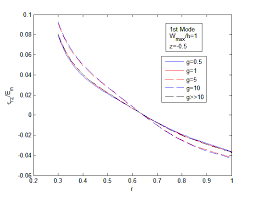The image above represents dimensionless radius.

To compute for the dimensionless radius, two essential parameters are needed and these parameters are external radius (re) and well radius (rw).

The formula for calculating the dimensionless radius:

rD = re / rw

Where;

Let’s solve an example;
Given that the external radius is 18 and the well radius is 16.

This implies that;

re = External Radius = 18
rw = Well Radius = 16

rD = re / rw
rD = 18 / 16
rD = 1.125

Therefore, the dimensionless radius is 1.125.

re = rD x rw

Where;

Let’s solve an example;
Given that the dimensionless radius is 9 and the well radius is 20.

This implies that;

rD = Dimensionless Radius = 9
rw = Well Radius = 20

re = rD x rw
re = 9 x 20
re = 180

Therefore, the external radius is 180.

rw = re / rD

Where;

Let’s solve an example;
Given that the dimensionless radius is 10 and the external radius is 90.

This implies that;

rD = Dimensionless Radius = 10
re = External Radius = 90

rw = re / rD
rw = 90 / 10
rw = 9

Therefore, the well radius is 9.

Nickzom Calculator – The Calculator Encyclopedia is capable of calculating the dimensionless radius.

To get the answer and workings of the dimensionless radius using the Nickzom Calculator – The Calculator Encyclopedia. First, you need to obtain the app.

You can get this app via any of these means:

To get access to the professional version via web, you need to register and subscribe for NGN 1,500 per annum to have utter access to all functionalities.
You can also try the demo version via https://www.nickzom.org/calculator

Apple (Paid) – https://itunes.apple.com/us/app/nickzom-calculator/id1331162702?mt=8
Once, you have obtained the calculator encyclopedia app, proceed to the Calculator Map, then click on Petroleum under EngineeringNow, Click on Well Testing under Petroleum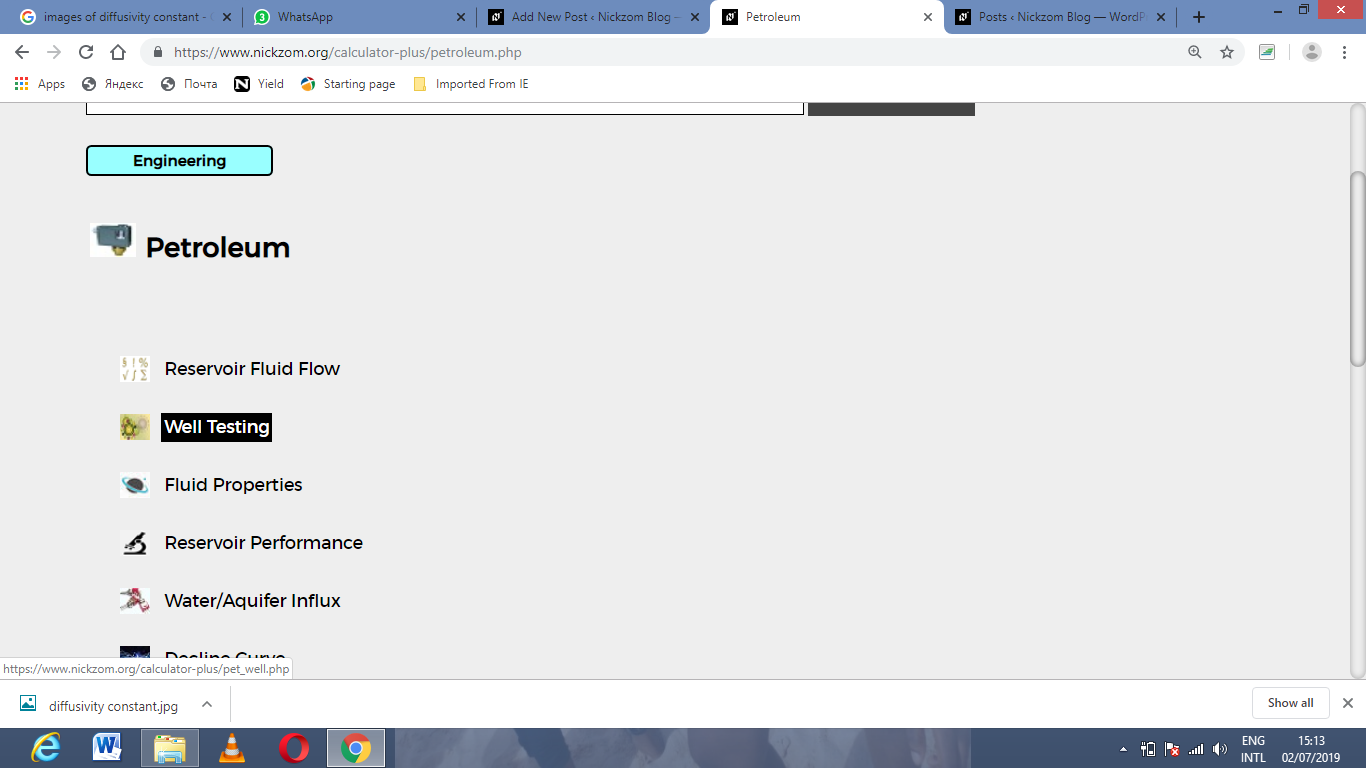Now, Click on Dimensionless Radius under Well Testing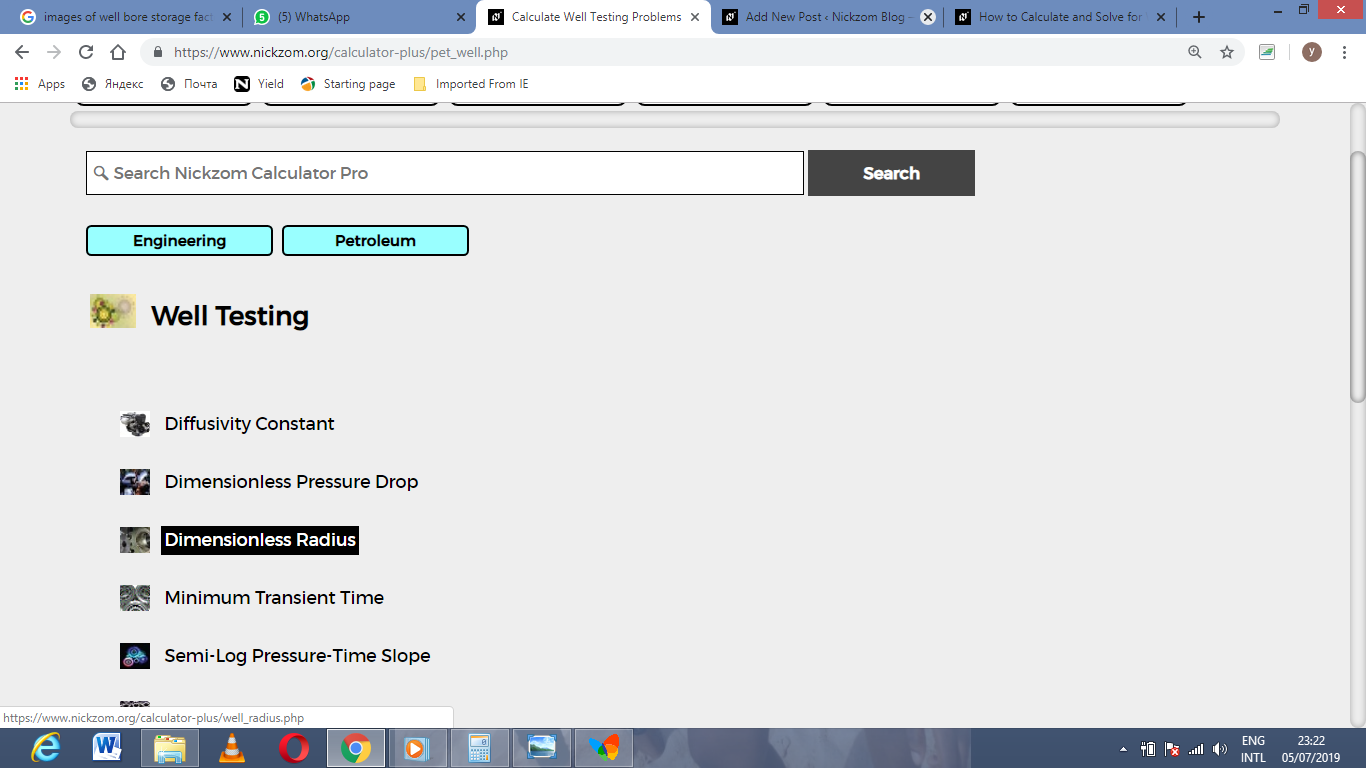The screenshot below displays the page or activity to enter your values, to get the answer for the dimensionless radius according to the respective parameters which are the external radius (re) and well radius (rw).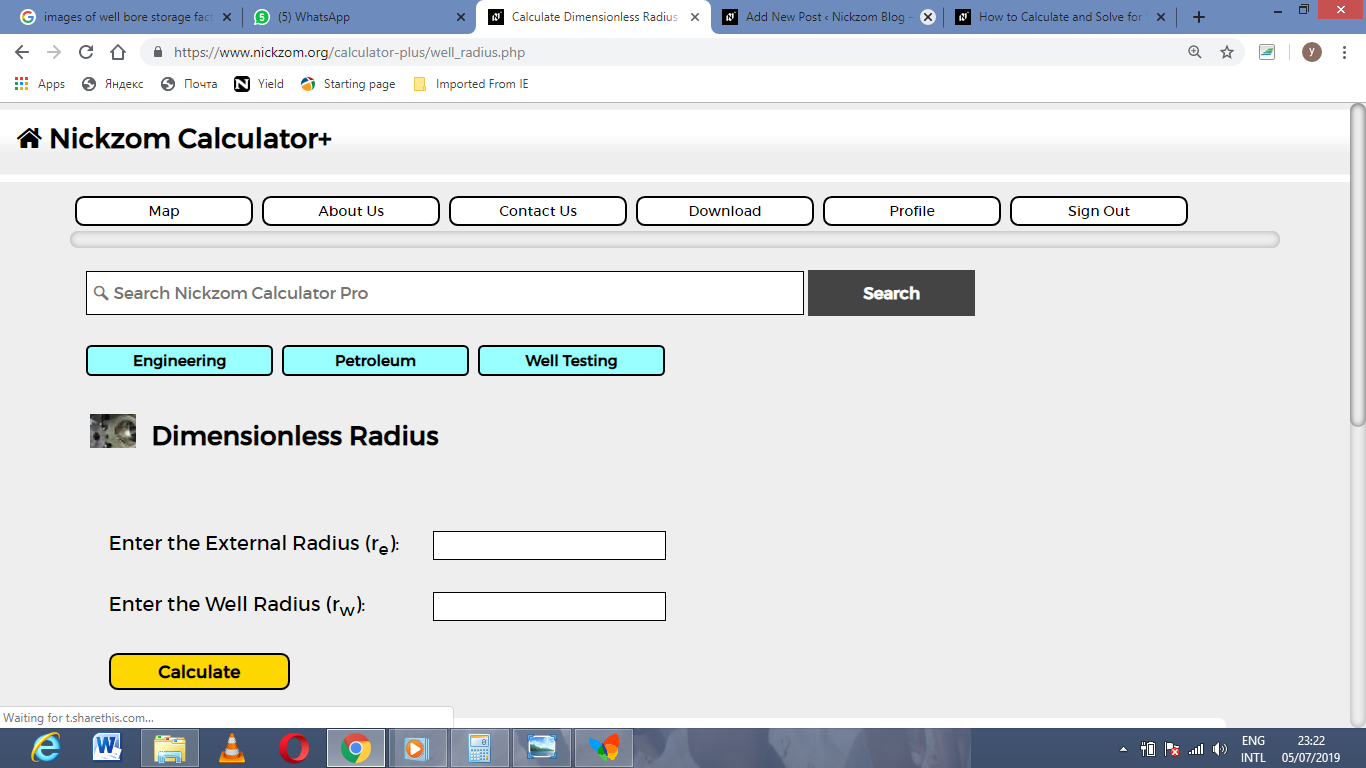Now, enter the values appropriately and accordingly for the parameters as required by the external radius (re) is 18 and well radius (rw) is 16.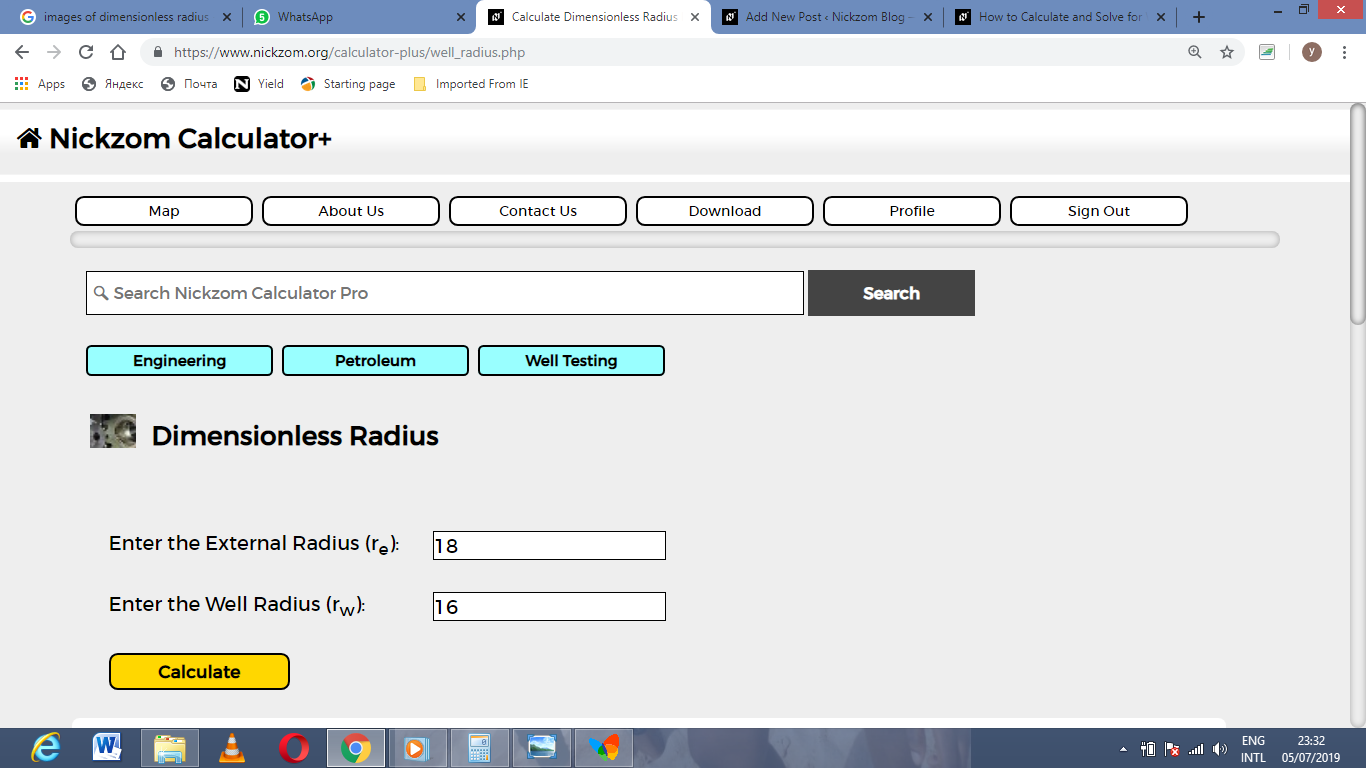Finally, Click on Calculate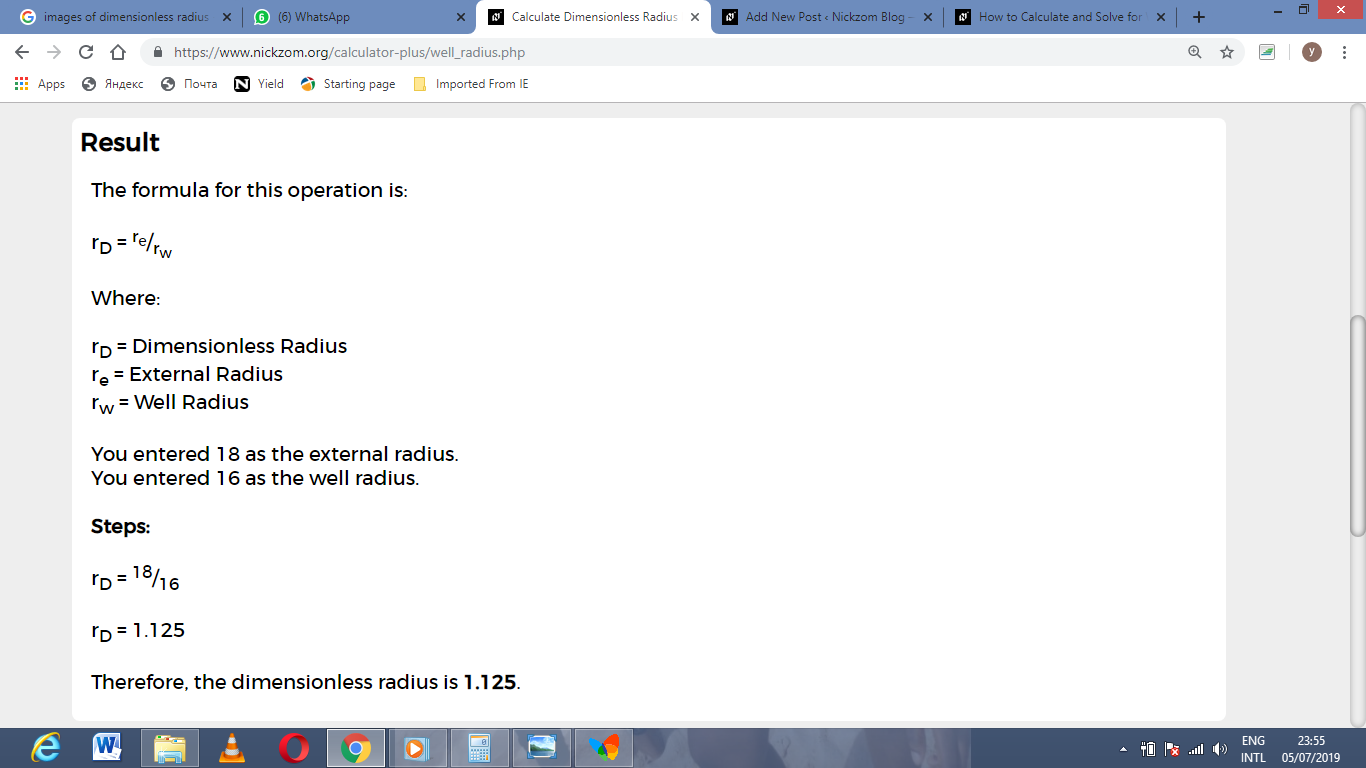As you can see from the screenshot above, Nickzom Calculator– The Calculator Encyclopedia solves for the dimensionless radius and presents the formula, workings and steps too.